# Image Processing 101: Color Space Conversion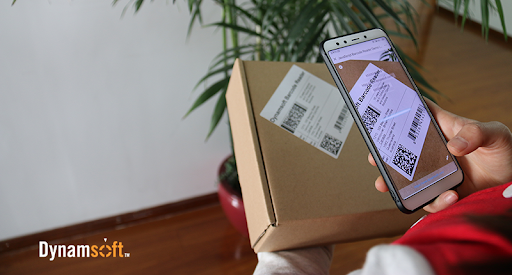In the last post, we discussed a few common color models, specifically RGB, HSV, and YUV. A color model is an abstract mathematical model that describes how colors can be represented as a set of numbers. In this post, we will look at how to convert them to grayscale. Grayscale is a range of gray shades from white to black, as used in a monochrome display or printout. Grayscale images are most commonly used in image processing because smaller data enables developers to do more complex operations in a shorter time.

1. ## Color to Grayscale Conversion

### 1.1 RGB to Grayscale

There are a number of commonly used methods to convert an RGB image to a grayscale image such as average method and weighted method.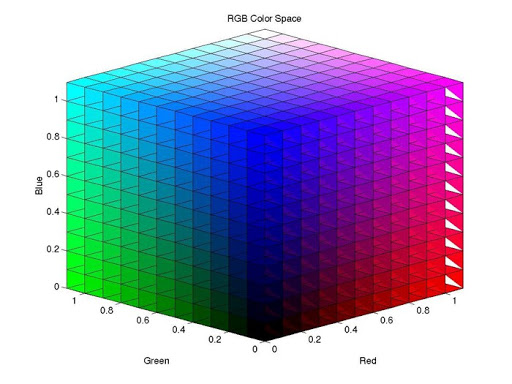#### Average Method

The Average method takes the average value of R, G, and B as the grayscale value.

Grayscale = (R + G + B ) / 3.

Theoretically, the formula is 100% correct. But when writing code, you may encounter uint8 overflow error — the sum of R, G, and B is greater than 255. To avoid the exception, R, G, and B should be calculated respectively.

Grayscale = R / 3 + G / 3 + B / 3.

The average method is simple but doesn’t work as well as expected. The reason being that human eyeballs react differently to RGB. Eyes are most sensitive to green light, less sensitive to red light, and the least sensitive to blue light. Therefore, the three colors should have different weights in the distribution. That brings us to the weighted method.

#### The Weighted Method

The weighted method, also called luminosity method, weighs red, green and blue according to their wavelengths. The improved formula is as follows:

Grayscale  = 0.299R + 0.587G + 0.114B### 1.2 YUV to Grayscale

YUV is a color encoding system used for analog television. The YUV color model represents the human perception of color more closely than the standard RGB model used in computer graphics hardware and is more size-efficient.

#### RGB to YUV Conversion

Y = 0.299R + 0.587G + 0.114B

U’= (B-Y)*0.565

V’= (R-Y)*0.713

#### The Grayscale Value

The formula for converting RGB to Y is the same as the formula for converting RGB to grayscale. Therefore:

Grayscale  = Y

### 1.3 HSV to Grayscale

HSV (hue, saturation, value)  and HSL (hue, saturation, lightness or luminance) are transformations of a Cartesian RGB color space.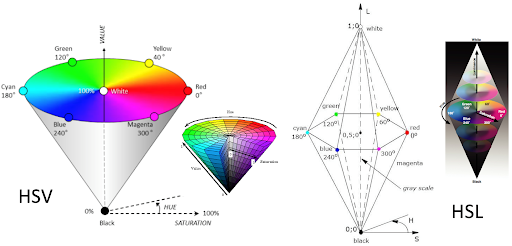To convert HSV to grayscale, we first need to convert HSV to RGB and then convert the RGB triple to a grayscale value.

#### HSV to RGB conversion

When 0 ≤ H < 360, 0 ≤ S ≤ 1 and 0 ≤ V ≤ 1:

C = V × S

X = C × (1 – |(H / 60°) mod 2 – 1|)

m = VC

(R,G,B) = ((R‘+m)×255, (G‘+m)×255, (B‘+m)×255)

#### The Grayscale Value

Grayscale  = 0.299R + 0.587G + 0.114B

1. ## Binarization: Grayscale to black/white Conversion

Binarization converts a grayscale image to a black/white image. This transformation is useful in detecting blobs and further reduces the computational complexity. The critical task is to find a suitable threshold. There are two main methods:

• Local thresholding — calculates the threshold pixel by pixel
• Global thresholding — calculates the threshold once for all pixels

The images below show an example of before and after binarization.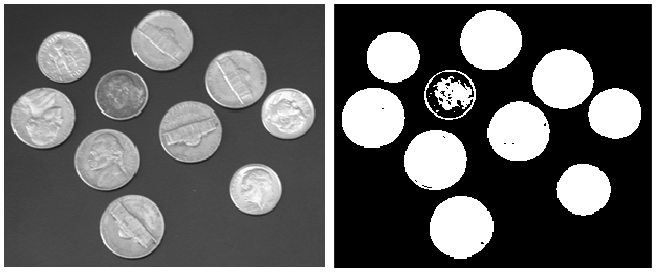### 2.1 Local Thresholding Method

With local thresholding method, a threshold is calculated at each pixel, which depends on some local statistics such as mean, range, and the variance of the pixel neighborhood. The image is divided into several sub-blocks and the distribution of gray-value in each block was analyzed.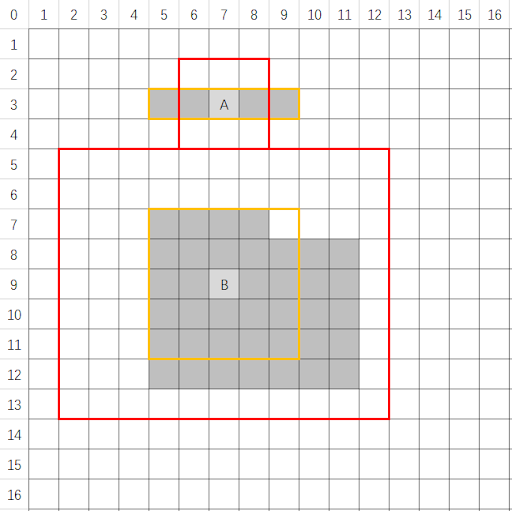### 2.2 Global Thresholding Method

The global thresholding method take advantage of the image histogram. The image histogram is a type of statistical graph with grayscale value on the x-axis and the number of pixels for each grayscale on the y-axis.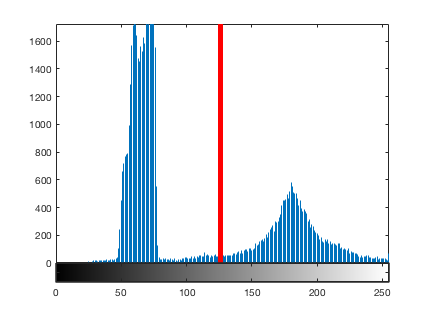Image histogram can be used to automatically determine the value of the threshold to be used for converting a grayscale image to a binary image. The basic idea is to find a point between the peak of the foreground pixel values and the peak of the background pixel values. If the intensity level of a pixel is smaller than the threshold, the pixel is set to black (grayscale = 0), otherwise, it is set to white (grayscale = 255). The threshold serves as a dividing line.

To learn more about imaging fundamentals, read the first article in the Image Processing 101 Series: What is an Image